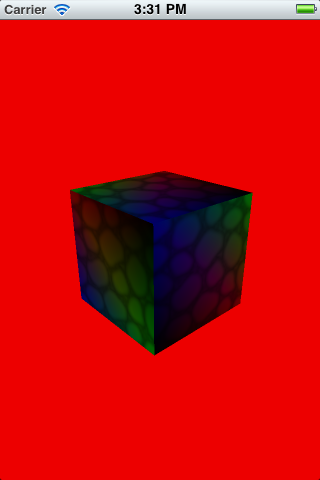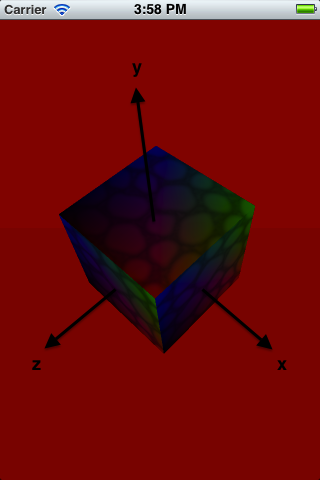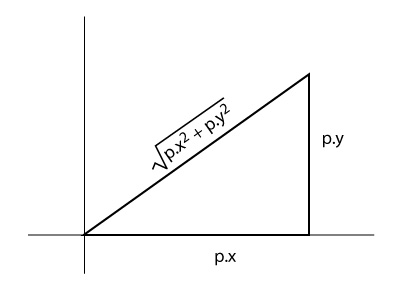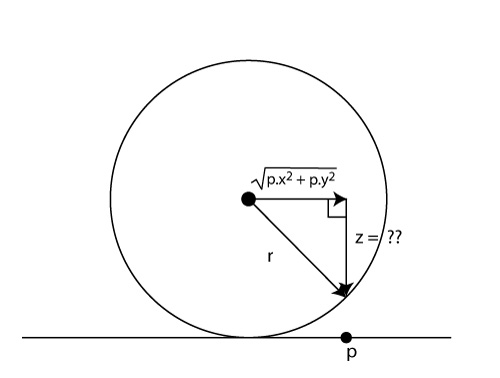# How To Rotate a 3D Object Using Touches with OpenGL

This is a blog post by site administrator Ray Wenderlich, an independent software developer and gamer. In this tutorial, you will learn how to rotate a 3D object with touches on iOS with OpenGL ES 2.0 and GLKit. We’ll start out simple and show you how you can rotate a 3D object by rotating a […]

#### Version

• Other, Other, Other

This is a blog post by site administrator Ray Wenderlich, an independent software developer and gamer.

In this tutorial, you will learn how to rotate a 3D object with touches on iOS with OpenGL ES 2.0 and GLKit.

We’ll start out simple and show you how you can rotate a 3D object by rotating a fixed amount along the x or y axis as the user drags. Then we’ll show you a more advanced technique using quaternions.

This tutorial will pick up with the sample project from the Beginning OpenGL ES 2.0 with GLKit Tutorial, so download it if you haven’t already.

Please keep in mind that I am learning this myself as I go and my math is quite rusty, so excuse me if I make any mistakes or don’t explain things quite right. If anyone has better or more correct ways to explain things, please chime in!

Without further ado, let’s get rotating!

## Simple Rotation: Attempt 1

Start by running the sample project, and you’ll see that the cube is already rotating at a fixed amount:If you open HelloGLKitViewController.m and find the update method, you can find the lines of code that are performing the fixed rotation:

```_rotation += 90 * self.timeSinceLastUpdate;
modelViewMatrix = GLKMatrix4Rotate(modelViewMatrix, GLKMathDegreesToRadians(25), 1, 0, 0);
modelViewMatrix = GLKMatrix4Rotate(modelViewMatrix, GLKMathDegreesToRadians(_rotation), 0, 1, 0);
```

Our goal is to replace this so that the user can rotate the cube by dragging the mouse, instead of having the rotation fixed.

Let’s make an initial attempt by moving the rotation matrix to an instance variable, and modifying it as the user drags. Make the following changes to HelloGLKitViewController.m:

```// Add to HelloGLKitViewController private variables
GLKMatrix4 _rotMatrix;

// Add to bottom of setupGL
_rotMatrix = GLKMatrix4Identity;

// In update, replace the 3 rotation lines shown in the previous snippet with this
modelViewMatrix = GLKMatrix4Multiply(modelViewMatrix, _rotMatrix);

// Remove everything inside touchesBegan
- (void)touchesBegan:(NSSet *)touches withEvent:(UIEvent *)event {

}

// Add new touchesMoved method
- (void)touchesMoved:(NSSet *)touches withEvent:(UIEvent *)event {

UITouch * touch = [touches anyObject];
CGPoint location = [touch locationInView:self.view];
CGPoint lastLoc = [touch previousLocationInView:self.view];
CGPoint diff = CGPointMake(lastLoc.x - location.x, lastLoc.y - location.y);

float rotX = -1 * GLKMathDegreesToRadians(diff.y / 2.0);
float rotY = -1 * GLKMathDegreesToRadians(diff.x / 2.0);

GLKVector3 xAxis = GLKVector3Make(1, 0, 0);
_rotMatrix = GLKMatrix4Rotate(_rotMatrix, rotX, xAxis.x, xAxis.y, xAxis.z);
GLKVector3 yAxis = GLKVector3Make(0, 1, 0);
_rotMatrix = GLKMatrix4Rotate(_rotMatrix, rotY, yAxis.x, yAxis.y, yAxis.z);

}
```

OK, so here we’re initializing the rotation matrix to the identity (no change). As the user drags, we use GLKMatrix4Rotate to rotate the cube a number of degrees. For every pixel the user drags, we rotate the cube 1/2 degree.

Remember that the x-axis is horizontal across the screen, and the y-axis is vertical. So when the user drags from left to right, we actually want to rotate around the y axis (rotY), and vice versa.

Compile and run, and you’ll notice that the cube seems to rotate OK at the beginning, but after a while it doesn’t behave the way you expect and starts rotating according to strange diagonal directions. What’s going on?

## Simple Rotation: Attempt 2

When you apply a transform to an object, you can think of it as moving the coordinate system of the object to a new space in the world.

To see what I mean, run the app and swipe from the upper right of the cube to about the middle of the cube. You have effectively modified the coordinate system of the object to be situated in a different world space as follows:The _rotMatrix is the transform that moves the object to this new space. Since our code is modifying the _rotMatrix as the user swipes, when we tell it to rotate around the x or y axis, it is rotating around the x or y axis in object space.

Try it out for yourself: make another swipe from right to left (careful not to move up or down), and you will see how it rotates around the new y-axis.

But what we really want to do is rotate around the world space x-axis and y-axis, not the object space x-axis and y-axis. But how can we figure out what the world space x-axis and y-axis will be in this new coordinate system?

The answer is simple: if _rotMatrix converts an object vector to where it should be in the world space, the inverse of _rotMatrix converts a world vector to where it should be in object space. You can see this through these equations:

```_rotMatrix * object vector = world vector
```

Multiply both sides by (_rotMatrix)-1 (the inverse of _rotMatrix), and you get:

```(_rotMatrix)-1 * _rotMatrix * object vector = (rotMatrix-1) * world vector
```

Since (_rotMatrix)-1 * _rotMatrix = 1, you get:

```object vector = (_rotMatrix)-1 * world vector
```

Let’s try this out! Modify touchesMoved to the following:

```- (void)touchesMoved:(NSSet *)touches withEvent:(UIEvent *)event {

UITouch * touch = [touches anyObject];
CGPoint location = [touch locationInView:self.view];
CGPoint lastLoc = [touch previousLocationInView:self.view];
CGPoint diff = CGPointMake(lastLoc.x - location.x, lastLoc.y - location.y);

float rotX = -1 * GLKMathDegreesToRadians(diff.y / 2.0);
float rotY = -1 * GLKMathDegreesToRadians(diff.x / 2.0);

bool isInvertible;
GLKVector3 xAxis = GLKMatrix4MultiplyVector3(GLKMatrix4Invert(_rotMatrix, &isInvertible),
GLKVector3Make(1, 0, 0));
_rotMatrix = GLKMatrix4Rotate(_rotMatrix, rotX, xAxis.x, xAxis.y, xAxis.z);
GLKVector3 yAxis = GLKMatrix4MultiplyVector3(GLKMatrix4Invert(_rotMatrix, &isInvertible),
GLKVector3Make(0, 1, 0));
_rotMatrix = GLKMatrix4Rotate(_rotMatrix, rotY, yAxis.x, yAxis.y, yAxis.z);

}
```

Compile and run, and now it rotates as expected!

## Arcball Rotation with Quaternions: Overview

Another popular way to handle rotating objects in 3D space is via the arcball rotation method popularized in a paper by Ken Shoemake.

Let me give you a brief overview of how arcball rotation works:

1. Map to sphere. Create a virtual sphere around the object you are trying to rotate. When the user touches, you figure out the closest point on the sphere corresponding to the touch (start), and similarly then the touch moves (current).
2. Calculate current rotation. To rotate a point on the sphere from start to current, you can think of it as a rotation of some degrees along some axis. Calculate this rotation/axis from the start/current positions.
3. Update overall rotation. Update the overall rotation of the object with the current rotation in step 3.

Step 3 is where the magic of a mathematical concept called quaternions comes in. I’m not going to discuss the math behind them here, but I will point out three high level properties of quaternions:

1. Quaternions = axis + angle of rotation. Quaternions can represent an axis and an angle of rotation around that axis. I.e. a quaternion can represent any rotation.
2. Multiply quaternions = combine rotations. If you multiply two quaternions, they represent the combination of the rotations they represent.
3. Can convert quaternions to matrices. Through some math (or a handy GLKit function) you can convert a quaternion to a rotation matrix.

One of the nice things about using GLKit is you can use quaternions without having to understand the mathematical equations behind how they work. You can use functions like:

• GLKQuaternionMakeWithAngleAndVector3Axis: Create a quaternion given an axis and an angle of rotation
• GLKQuaternionMultiply: Multiply one quaternion via another (combining the rotations)
• GLKMatrix4MakeWithQuaternion: Convert quaternion to a rotation matrix

If you’re still a little confused to how this all works, don’t worry – we’re going to go through this step by step in the next few sections!

1) Map to Sphere.

Imagine we have a virtual sphere surrounding our object, with a radius of 1/3 the screen width. The center of the sphere is the center of the object we’re rotating. We want to let the user “grab and drag” this sphere to rotate the object.

So the first step is to figure out how to convert a 2D touch point to a point on a virtual 3D sphere surrounding the object.

Here’s the easiest way I found to visualize how to figure this out. First, let’s take a front view of where the user taps (from the center of the object):We know the x position the user taps, and the y position the user taps, so we can figure out the length of the hypotenuse via the Pythagorean Theorem.

Now imagine we’re looking from top down instead, with the screen being the line at the bottom:We now know the vector going from the center of the sphere to where the user tapped along the x and y axis, but we do not know the z coordinate.

However, we do know that the z coordinate needs to intersect with the sphere, and if you draw a line from the center of the sphere to any point, it has a length of the radius of the sphere (by definition).

Therefore, we have another right triangle and can use the pythagorean theorem to solve! We get:

```r^2 = (sqrt(p.x^2 + p.y^2))^2 + z^2
r^2 = (p.x^2 + p.y^2) + z^2
r^2 - (p.x^2 + p.y^2) = z^2
```

The only tricky bit is if the user clicks outside the radius of the sphere. If this happens, we’ll use the closest point on the sphere we can find instead.

Let’s see what this looks like in code! Add the following method right before touchesBegan:

```- (GLKVector3) projectOntoSurface:(GLKVector3) touchPoint
{
float radius = self.view.bounds.size.width/3;
GLKVector3 center = GLKVector3Make(self.view.bounds.size.width/2, self.view.bounds.size.height/2, 0);
GLKVector3 P = GLKVector3Subtract(touchPoint, center);

// Flip the y-axis because pixel coords increase toward the bottom.
P = GLKVector3Make(P.x, P.y * -1, P.z);

float radius2 = radius * radius;
float length2 = P.x*P.x + P.y*P.y;

if (length2 <= radius2)
P.z = sqrt(radius2 - length2);
else
{
P.x *= radius / sqrt(length2);
P.y *= radius / sqrt(length2);
P.z = 0;
}

return GLKVector3Normalize(P);
}
```

This implements the same math we discussed above. Note we also normalize the vector at the end, because when calculating rotations it's the direction that matters more than the length.

Now let's start moving it. Make the following changes to HelloGLKitViewController.m:

```// Add to the private interface
GLKVector3 _anchor_position;
GLKVector3 _current_position;

// Add to bottom of touchesBegan
UITouch * touch = [touches anyObject];
CGPoint location = [touch locationInView:self.view];

_anchor_position = GLKVector3Make(location.x, location.y, 0);
_anchor_position = [self projectOntoSurface:_anchor_position];

_current_position = _anchor_position;

// Add to bottom of touchesMoved
_current_position = GLKVector3Make(location.x, location.y, 0);
_current_position = [self projectOntoSurface:_current_position];
```

So far so good! We now have normalized vectors pointing to the start and end points on the sphere corresponding to the user's mouse movements. Now let's use them to calculate an axis and angle of rotation!

2) Calculate current rotation.

To calculate the axis and angle of rotation, we can use our friends the dot product and the cross product.

If you are a little rusty as to what these do, I highly recommend reading the article Linear Algebra for Game Developers by David Rosen. It does a great job of introducing these concepts in a simple and easy to understand way.

But if you can't be bothered, I'll give a brief summary here:

• The cross product allows you to give two vectors, and it will give you the vector perpendicular (90 degrees) to both vectors. This will be the axis of rotation, now we just need to figure out the amount to rotate from one vector to the other along this axis.
• Long story short, you can use the dot product to help determine the angle between two vectors. The angle is acos(dot(A, B)) if A and B are unit vectors. And ours are unit vectors - remember how we normalized them at the end of projectOntoSurface.

Once we determine the axis and angle, we can create a quaternion to store them using the handy GLKit GLKQuaternionMakeWithAngleAndVector3Axis function.

Let's try it out! Make the following changes to HelloGLKitViewController.m:

```// Add new method above touchesBegan
- (void)computeIncremental {

GLKVector3 axis = GLKVector3CrossProduct(_anchor_position, _current_position);
float dot = GLKVector3DotProduct(_anchor_position, _current_position);
float angle = acosf(dot);

GLKQuaternion Q_rot = GLKQuaternionMakeWithAngleAndVector3Axis(angle * 2, axis);
Q_rot = GLKQuaternionNormalize(Q_rot);

// TODO: Do something with Q_rot...

}

// Call it at end of touchesEnded
[self computeIncremental];
```

OK awesome, we now have a quaternion representing how we can rotate the object from the start touch point to the current touch point (mapped to the sphere), now what?

3) Update overall rotation.

For the final step, we need to apply the rotation to the object. To do this we need to track two rotations: the original rotation of the object, and the current (temporary) rotation as the user drags their finger.

This is pretty simple so let's just dive into code. Make the following changes to HelloGLKitViewController.m:

```// Add new variables to private interface
GLKQuaternion _quatStart;
GLKQuaternion _quat;

// Initialize them at bottom of setupGL
_quat = GLKQuaternionMake(0, 0, 0, 1);
_quatStart = GLKQuaternionMake(0, 0, 0, 1);

// Set _quat at bottom of computeIncremental
_quat = GLKQuaternionMultiply(Q_rot, _quatStart);

// Set _quatStart at bottom of touchesBegan
_quatStart = _quat;

// In update, replace GLKMatrix4Multiply(modelViewMatrix, rotation) line with this:
GLKMatrix4 rotation = GLKMatrix4MakeWithQuaternion(_quat);
modelViewMatrix = GLKMatrix4Multiply(modelViewMatrix, rotation);
```

Here _quatStart represents the original rotation of the object (before the user starts dragging), and _quat is the current (temporary) rotation.

At the end of computeIncremental, we multiply the original rotation with the current rotation (i.e. the amount to rotate from the start to the current touch point).

In update, we convert the quaternion into a rotation matrix, and apply it to the model view matrix as usual.

Compile and run, and now you can rotate the cube with arcball rotation!

## Bonus: Alternative projectOntoSurface

Note how with the current implementation, if you drag outside the sphere it maps to the closest point on the sphere rather than continuing the rotation. To make the rotation continue instead, replace the else clause in projectOntoSurface with this:

```P.z = radius2 / (2.0 * sqrt(length2));
float length = sqrt(length2 + P.z * P.z);
P = GLKVector3DivideScalar(P, length);
```

## Bonus: More Fun with Quaternions

You might wonder what else you can do with quaternions. One cool thing they can do is provide an easy way to interpolate between two rotations over time.

To see what I mean, let's try it out and add some code to make our cube animate back to its original orientation if you double tap. Make the following changes to HelloGLKitViewController.m:

```// Add new private instance variables
BOOL _slerping;
float _slerpCur;
float _slerpMax;
GLKQuaternion _slerpStart;
GLKQuaternion _slerpEnd;

// Add to bottom of setupGL
UITapGestureRecognizer * dtRec = [[UITapGestureRecognizer alloc] initWithTarget:self action:@selector(doubleTap:)];
dtRec.numberOfTapsRequired = 2;
[self.view addGestureRecognizer:dtRec];

// Add new method
- (void)doubleTap:(UITapGestureRecognizer *)tap {

_slerping = YES;
_slerpCur = 0;
_slerpMax = 1.0;
_slerpStart = _quat;
_slerpEnd = GLKQuaternionMake(0, 0, 0, 1);

}

// Add inside update method, right before declaration of modelViewMatrix
if (_slerping) {

_slerpCur += self.timeSinceLastUpdate;
float slerpAmt = _slerpCur / _slerpMax;
if (slerpAmt > 1.0) {
slerpAmt = 1.0;
_slerping = NO;
}

_quat = GLKQuaternionSlerp(_slerpStart, _slerpEnd, slerpAmt);
}
```

When the user double taps, we set the start rotation to the current orientation (_quat), and the end rotation to the "identity" quaternion (no rotation at all).

Then inside update, if we are "sleeping" we figure out how far along the animation we are so far. Then we use the built-in GLKQuaternionSlerp method to come up with the appropriate rotation in-between slerpStart and slerpEnd, based on the current time.

Compile and run, rotate the object, and double tap to make it animate back to its original position. This technique is commonly used for keyframe animations on 3D objects and the like.

## Where To Go From Here?

Here is an example project with all of the code from the above tutorial.

Hopefully this tutorial was helpful to others who wanted to learn a little more about rotating 3D objects based on touches and brush up on some 3D math concepts.

If anyone has any questions, corrections, or better or easier ways to explain things, please join the forum discussion below!This is a blog post by site administrator Ray Wenderlich, an independent software developer and gamer.